Open In App
Related Articles
• Write an Interview Experience
• C++ Programming Language

# cin in C++

The cin object in C++ is an object of class iostream. It is used to accept the input from the standard input device i.e. keyboard. It is associated with the standard C input stream stdin. The extraction operator(>>) is used along with the object cin for reading inputs. The extraction operator extracts the data from the object cin which is entered using the keyboard.

Program 1:

Below is the C++ program to implement cin object:

## C++

 `// C++ program to demonstrate the``// cin object``#include ``using` `namespace` `std;`` ` `// Driver Code``int` `main()``{``    ``string s;`` ` `    ``// Take input using cin``    ``cin >> s;`` ` `    ``// Print output``    ``cout << s;`` ` `    ``return` `0;``}`

Input:Output:Program 2:

Multiple inputs using the extraction operators(>>) with cin. Below is the C++ program to take multiple user inputs:

## C++

 `// C++ program to illustrate the take``// multiple input``#include ``using` `namespace` `std;`` ` `// Driver Code``int` `main()``{``    ``string name;``    ``int` `age;`` ` `    ``// Take multiple input using cin``    ``cin >> name >> age;`` ` `    ``// Print output``    ``cout << ``"Name : "` `<< name << endl;``    ``cout << ``"Age : "` `<< age << endl;`` ` `    ``return` `0;``}`

Input: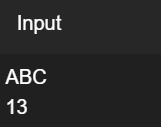Output: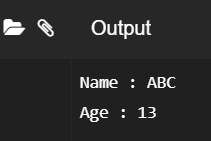The cin can also be used with some member functions which are as follows:

### cin.getline(char *buffer, int N):

It reads a stream of characters of length N into the string buffer, It stops when it has read (N – 1) characters or it finds the end of the file or newline character(\n). Below is the C++ program to implement cin.getline():

## C++

 `// C++ program to illustrate the use``// of cin.getline``#include ``using` `namespace` `std;`` ` `// Driver Code``int` `main()``{``    ``char` `name;`` ` `    ``// Reads stream of 3``    ``// characters``    ``cin.getline(name, 3);`` ` `    ``// Print output``    ``cout << name << endl;`` ` `    ``return` `0;``}`

Input: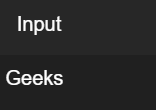Output: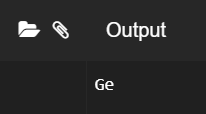### cin.get(char& var):

It reads an input character and stores it in a variable.  Below is the C++ program to implement cin.get():

## C++

 `// C++ program to illustrate the use``// of cin.get()``#include ``using` `namespace` `std;`` ` `// Driver Code``int` `main()``{``    ``char` `ch;``    ``cin.get(ch, 25);`` ` `    ``// Print ch``    ``cout << ch;``}`

Input:Output: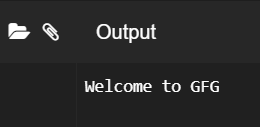Reads a stream of characters of length N. Below is the C++ program to implement cin.read():

## C++

 `// C++ program to illustrate the use``// of cin.read()``#include ``using` `namespace` `std;`` ` `// Driver Code``int` `main()``{``    ``char` `gfg;`` ` `    ``// Reads stream of characters``    ``cin.read(gfg, 10);`` ` `    ``// Print output``    ``cout << gfg << endl;`` ` `    ``return` `0;``}`

Input:Output: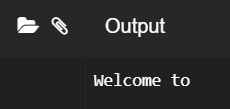### cin.ignore():

It ignores or clears one or more characters from the input buffer. Below is the C++ program to implement cin.ignore():

## C++

 `// C++ program to illustrate the use``// of cin.ignore()``#include `` ` `// used to get stream size``#include `` ` `// used to get numeric limits``#include ``using` `namespace` `std;`` ` `// Driver Code``int` `main()``{``    ``int` `x;``    ``char` `str;``    ``cout << ``"Enter a number andstring:\n"``;``    ``cin >> x;`` ` `    ``// clear buffer before taking``    ``// new line``    ``cin.ignore(numeric_limits::max(), ``'\n'``);`` ` `    ``// Input a string``    ``cin.getline(str, 80);``    ``cout << ``"You have entered:\n"``;``    ``cout << x << endl;``    ``cout << str << endl;`` ` `    ``return` `0;``}`

Input:Output: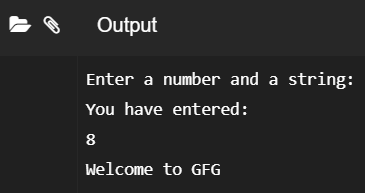Explanation: In the above program if cin.ignore() has not been used then after entering the number when the user presses the enter to input the string, the output will be only the number entered. The program will not take the string input. To avoid this problem cin.ignore() is used, this will ignore the newline character.